• Nota de Estudos
• Rever TópicosBatjargal P. 0 0 Fraction: # of possibilities meeting my condition over # of equally likely possibilities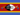Samkeliso R S. 0 0 not clear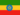Henock A. 0 0 great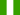Juliet N. 0 0 some answers are not clear enough but some are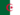Massinissa G. 0 0 interesting.Corey N. 0 0 Activities are childish.Wilfred O. 1 0 I kind of disagree with the answer given in the last button of "Probability with equally likely outcomes" above. The question was to choose a single-digit factor of six. I was thinking this... Single digits: 1, 2, 3, 4, 5, 6, 7, 8, 9. i.e S = 9. Factors of 6: 2, and 3. i.e n = 2 Probability = n/s = 2/9. The answer given is 2/5, can anyone explain this to me?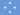June A. 0 0 There is a problem with the link to the Quiz! cannot open the quiz...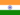Hitesh K. 0 0 fine easy to understandHitesh K. 0 0 can wecalculate probability of two events occuring separately
• Text Version### Você recebeu uma nova notificação

Clique aqui para visualizar todos eles